# Power BI – Year over Year (YOY) Reports using SAMEPERIODLASTYEAR

In Power BI, we may want to compare periods with our data to create reports such as year over year comparisons. Power BI offers several DAX time intelligence functions. In this post we will look at SAMEPERIODLASTYEAR.

Let’s say we have an Orders table that contains orders for the last 2 years. The table contains an Order Date and a Ship Date: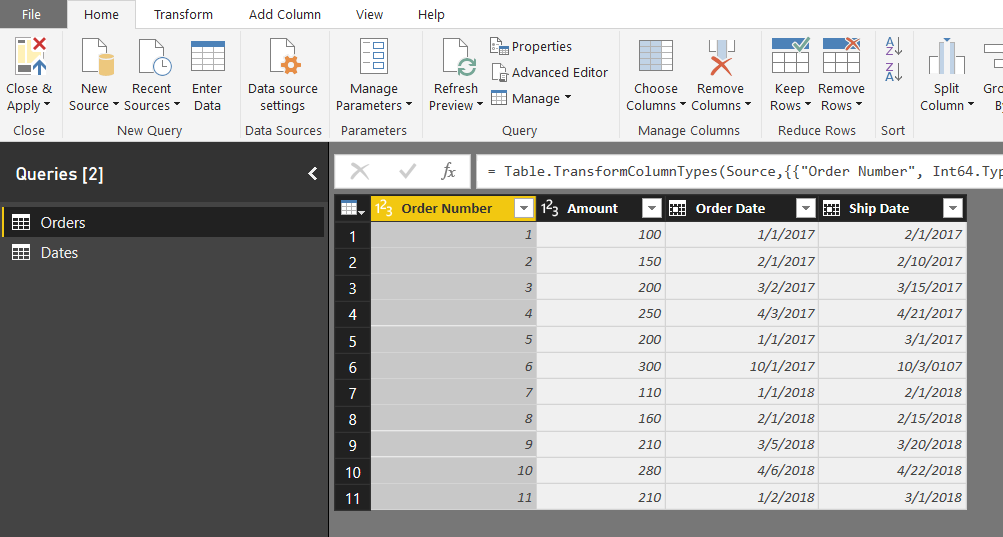We also have a standard Dates table.

Now let’s say we want to compare this year’s sales to last year’s sales.

First, create a measure for the total sales: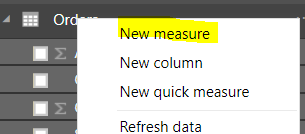Formula: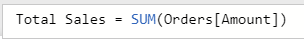`Total Sales = SUM('Orders'[Amount])`

We can see, the total sales amount is 2170: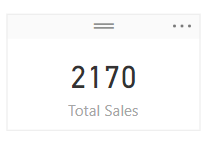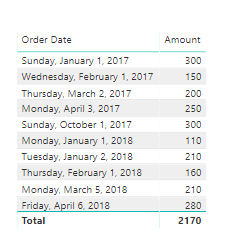Now let’s use SAMEPERIODLASTYEAR to create a new measure. This will be based on the measure we just created, Total Sales, and we will use the SAMEPERIODLASTYEAR function based on dates: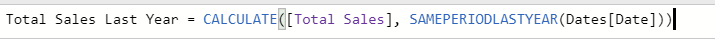`Total Sales Last Year = CALCULATE([Total Sales], SAMEPERIODLASTYEAR(Dates[Date]))`

Now let’s drag across the Date from the date table, with the Total Sales and Total Sales Last Year measures. You can see we are comparing each day’s current year and previous year, for example, on February 1st, there was an amount of 160 this year and 150 last year: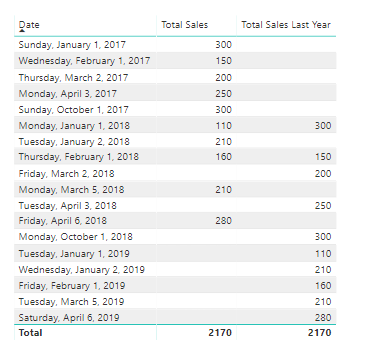Now, if we want to filter this so that it only shows results before today, we can use:

`Total Sales Last Year = CALCULATE(CALCULATE([Total Sales], SAMEPERIODLASTYEAR(Dates[Date])), FILTER(Dates, Dates[Date] < TODAY()))`

and

`Total Sales = CALCULATE(SUM(Orders[Amount]), FILTER(Dates, Dates[Date] < TODAY()))`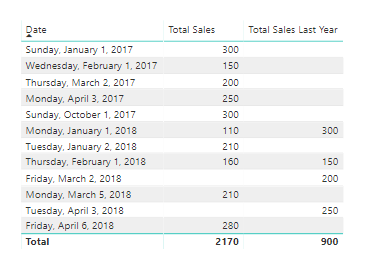We can create count measures to see the comparisons as well:

`Count Sales = CALCULATE(COUNT(Orders[Order Number]), FILTER(Dates, Dates[Date] < TODAY()))`

And:

`Count Sales Last Year = CALCULATE(CALCULATE([Count Sales], SAMEPERIODLASTYEAR(Dates[Date])), FILTER(Dates, Dates[Date] < TODAY()))`

Produces below. You can see on January 1st 2018, there was 1 sale, and 2 in the previous period (January 1st, 2017):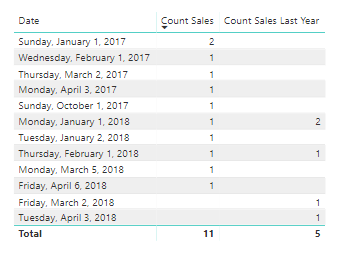If you want to compare additional date relationships, you can do the following. For example, we can add a new field called Total Sales by Ship Date, which uses the field Ship Date:

```Total Sales by Ship Date = CALCULATE(CALCULATE (
SUM ( Orders[Amount] ),
USERELATIONSHIP (
Orders[Ship Date],
Dates[Date]
)
)
, FILTER(Dates, Dates[Date] < TODAY()))```

And add a Total Sales by Ship Date Last Year using SAMEPERIODLASTYEAR:

`Total Sales by Ship Date Last Year = CALCULATE(CALCULATE([Total Sales by Ship Date], SAMEPERIODLASTYEAR(Dates[Date])), FILTER(Dates, Dates[Date] < TODAY()))`

This produces: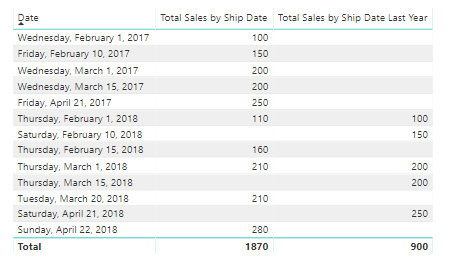If you would like to replace the blanks with 0, you can use the ISBLANK() DAX function.Carl de Souza is a developer and architect focusing on Microsoft Dynamics 365, Power BI, Azure, and AI.

### 7 Responses to Power BI – Year over Year (YOY) Reports using SAMEPERIODLASTYEAR

1. mike says:

Carl this phenomenal! I cannot tell you how long that I’ve been wrapped up in trying to figure this out! Thank you, thank you, thank you!

• Carl says:

Great Mike! Good luck with your project.

2. Rohit says:

Hi Carl,

I have a similar question but with a different data set, where the data is in vertical format and can’t be changed/ transposed as I have created charts using the same format. I need to calculate YoY % change for each KPI. I was wondering if this could be done using DAX formula:

Customer KPI unit Period Value
Verizon Total revenue USD million Q1/2016 29029
Verizon Total revenue USD million Q1/2017 27959
Verizon Capex USD million Q1/2016 10029
Verizon Capex USD million Q1/2017 8959
Bharti Total revenue USD million Q1/2016 34567
Bharti Total revenue USD million Q1/2017 33000
Bharti Capex USD million Q1/2016 15222
Bharti Capex USD million Q1/2017 13655

Thanks,

3. Sometimes someone just has the ability to explain something simply. I wasted 30 minutes on Youtube videos and 15 minutes on other posts.

And then this! Fantastic.. So well explained!

• Carl says:

Thanks Nic, I appreciate it!

4. Eghosa Obazee says:

Hi Carl,
Please can you help me with Year on Year (%) Change DAX Formula in Power BI.
I am trying to calculate (%) Change Year on Year, say Year 2013 – Year 2019.
Thanks.
Eghosa